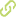# Zero forcing number of graphs Journalartikel### Abstract

• A subset S of initially infected vertices of a graph \$G\$ is called forcing if we can infect the entire graph by iteratively applying the following process. At each step, any infected vertex which has a unique uninfected neighbour, infects this neighbour. The forcing number of \$G\$ is the minimum cardinality of a forcing set in \$G\$. In the present paper, we study the forcing number of various classes of graphs, including graphs of large girth, \$H\$-free graphs for a fixed bipartite graph \$H\$, random and pseudorandom graphs.

• 2019

### Beitrag veröffentlicht in

• arXiv  Integrierende Ressource

• 33

• 95

• 115